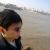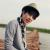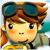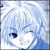## php中遍历多维数组的方法

booleanyong 发布于 2012/06/28 19:01

function arr_foreach (\$arr) {
if (!is_array (\$arr)) {
return false;
}
foreach (\$arr as \$key => \$val ) {
if (is_array (\$val)) {
arr_foreach (\$val);
} else {
\$str .= ','.\$val;
}
}
echo \$str;
}

\$firstarr = array('sdsdf','3332fsdf',

array(

'a','b','c',array(

array(33,3,4,5),'e','f',array(1,23,45)

)

)

);

//echo "<pre>";

arr_foreach(\$firstarr);

### 以下是问题补充：

@booleanyong：我的本意，是想把多维数组变成一维数组而已！ (2012/06/29 09:50)

0```function arr_foreach (\$arr) {
static \$data;
if (!is_array (\$arr)) {
return \$data;
}
foreach (\$arr as \$key => \$val ) {
if (is_array (\$val)) {
arr_foreach (\$val);
} else {
\$data[]=\$val;
}
}
return \$data;
}
\$data=arr_foreach(\$arr);```00#### 引用来自“飞晏”的答案

0#### 引用来自“飞晏”的答案

0```function arr_foreach (\$arr) {
static \$str;
if (!is_array (\$arr)) {
return false;
}
foreach (\$arr as \$key => \$val ) {

if (is_array (\$val)) {

arr_foreach (\$val);
} else {

\$str[] = \$val;
}
}
return \$str;
}```